# Algebra of Matrices

## What is Matrix

A matrix is a rectangular arrangement of numbers (real or complex) which may be represented as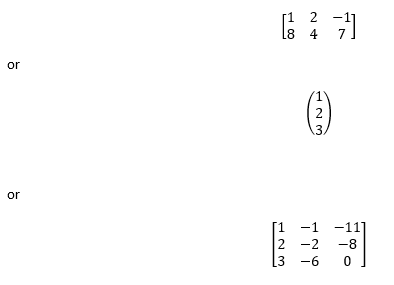1) matrix is enclosed by [ ] or ( ) or | |

2) Compact form the above matrix is represented by

[aij](m×n) or A = [aij]

3) Element of a Matrix :The numbers a11, a12… etc., in the above matrix are known as the element of the matrix, generally represented as aij, which denotes element in ith row and jth column.

4.Order of a Matrix: In above matrix has m rows and n columns, then A is of order m x n

## Types of Matrices

1) Row Matrix

A matrix having only one row and any number of columns is called a row matrix.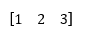2.Column Matrix

A matrix having only one column and any number of rows is called column matrix.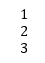3.Rectangular Matrix

A matrix of order m x n, such that m ≠ n, is called rectangular matrix.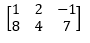4.Horizontal Matrix

A matrix in which the number of rows is less than the number of columns, is called a horizontal matrix

5.Vertical Matrix

A matrix in which the number of rows is greater than the number of columns, is called a vertical matrix.

6.Null/Zero Matrix

A matrix of any order, having all its elements are zero, is called a null/zero matrix. i.e., aij= 0,∀i,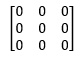7) Square Matrix

A matrix of order m x n, such that m = n, is called square matrix.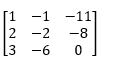8.Diagonal Matrix

A square matrix A= [aij](m×n), is called a diagonal matrix, if all the elements except those in the leading diagonals are zero, i.e., aij= 0 for i ≠ j. It can be represented as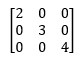9.Scalar Matrix

A square matrix in which every non-diagonal element is zero and all diagonal elements are equal, is called scalar matrix. i.e., in scalar matrix

aij= 0, for i ≠ j and aij= k, for i = j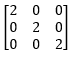## Equality of Matrices

Equal matrices have identical corresponding elements.

If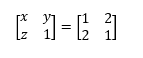Then

a=1,y=2,z=2

## Addition (and Subtraction) of Matrices

We can only add (or subtract) matrices if they have the same dimensions. That is, the two matrices must have the same number of rows and the same number of columns.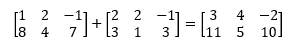Note: We started with two matrices, both having dimensions 2 × 3. Our answer was also a 2 × 3 matrix.

Let A, B, and C be m×n matrices.
 Commutativity A+ B =B+A Associativity (A+B)+C = A + (B+C) Existence of Identity A + O = A= O+ A Where O is the Null matrix Existence of Inverse A +(-A) =0 So -A is the additive inverse of A Cancellation A+ B= B +C A=C

## Identity Matrix

The Identity Matrix, written I, is a square matrix where all the elements are 0 except the principal diagonal which has all ones.

Here is the 2 × 2 identity matrix: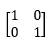Here is the 3 × 3 identity matrix: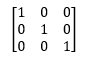## Scalar of  Multiplication/Division Matrix

Let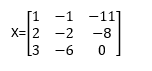Then Multiplication by scalar would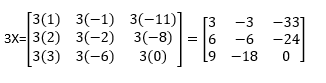1) So each element will be multiplied by the scalar

Similary for divison ,every element will be divided by the scalar

## Properties of Scalar Multiplication

 Let A and B be m×n matrices, p and q are scalar, then p(A+B) = pA + qB (p+q)A= pA + qA (pq)A=p(qL) =q(pA) (-p)A= -(pA) = p(-A)

## Multiplication Of Matrix

Two matrics can be multiplied  if the number of columns in the first matrix is the same as the number of rows in the second matrix

i.e,

1)  2X3  Matrix can be Multiplied with 3X [1…]

2) 3x2 Matrix can be Multiplied with 2X [1…]

3) 3x2 Matrix can not be Multiplied with [Any number except 2]X [1…]

In general

A =(x,y)  And B( y,z)  Then Multiplication of A and B is possible, the resultant matric would be C(x,z)

The element of C would be defined as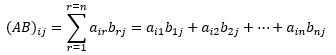We can say it this way. We work across the 1st row of the first matrix, multiplying down the 1st column of the second matrix, element by element. We add the resulting products. Our answer goes in position (AB)11   of the answer matrix.

We do a similar process for the 1st row of the first matrix and the 2nd column of the second matrix. The result is placed in position (AB)12

This is how multiplication works for (2 × 3)  and ( 3 ×2) Matrices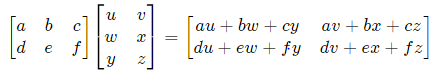Properties of Matrix Multiplication
 AB ≠ BA (AB)C= A(BC) A(B+C)=AB + AC (A+B)C=AC + BC ImA= A= AIn

## Transpose of Matrix

Let A =(aij) m×n  , then transpose of A is defined as n× m matrix such as

(AT)ij= aji  for all i=1,2,3….m;j=1,2,3….n

So AT  is obtained  from A by changing its rows into columns and columns into rows

Example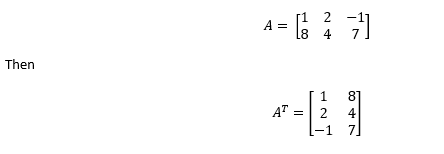## Properties of Transpose

 (AT)T=A (A+B)T=AT + BT (kA)T=kAT (AB)T=BTAT

## Why study the Matrix?

Applications of matrices are found in most scientific fields. In every branch of physics, including classical mechanics, optics, electromagnetism, quantum mechanics, and quantum electrodynamics, they are used to study physical phenomena, such as the motion of rigid bodies. In computer graphics, they are used to project a 3D model onto a 2 dimensional screen. In probability theory and statistics, stochastic matrices are used to describe sets of probabilities; for instance, they are used within the PageRank algorithm that ranks the pages in a Google search. Matrix calculus generalizes classical analytical notions such as derivatives and exponentials to higher dimensions.

## How to Solve Matrix Problem

1)  You need to remember the rules of addition ,subtraction, multiplication by scalar and Multiplication of Matrix

2) apply the principle and solve the questions

## Solved Examples

1)  Given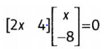Find the negative  value of x

Solution:

My Multiplication of Matrics (1×2)  and ( 2×1) ,we get

2x(x) +4(-8)  =0

2x2 -32=0

x= 4 or -4

So x =-4

2)  Given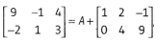Find the Matrix A

Solution

Given Matrix equation can  be written as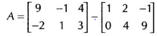Or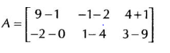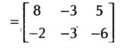3)  Given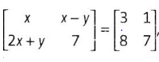Find the value of x and y

SolutionBy equality of Matrix

x=3

x-y=1 or y =x-1=2

## Related Topics

Go back to Class 12 Main Page using below links